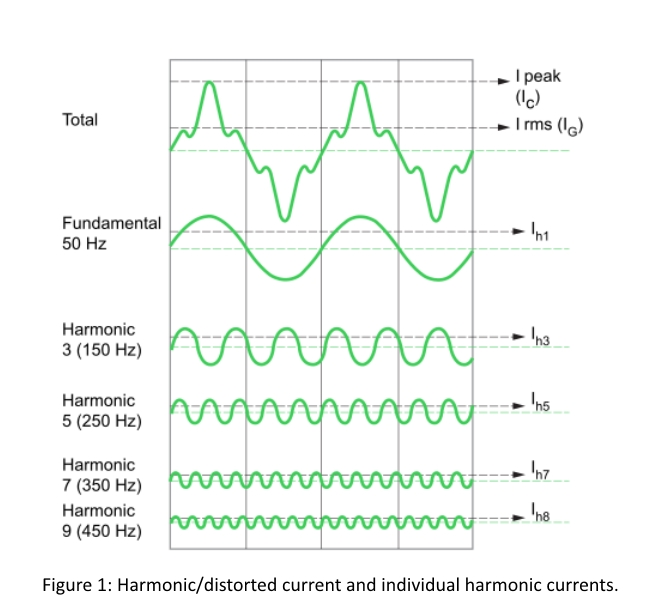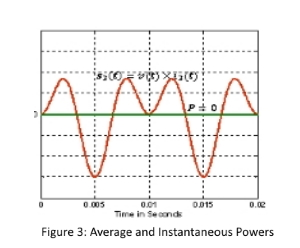# Knowledge Center

## Harmonics

Harmonics in the current or voltage are components at a multiple of fundamental frequency of the system. They are generated mainly due to turn on and off (switching) operation of power electronics-based system, for example, variable frequency drives-VFDs, phase-controlled rectifiers, choppers, battery chargers, televisions, printers, laptops, CFLs and so on.

In Fig. 1, the top waveform represents the distorted/harmonic current. Typically, Fast Fourier Transform (FFT) technique is used to decompose the distorted signal in to set of sinusoidal signals of different frequencies. The fundamental frequency (for example, 50Hz (India) or 60Hz (USA)) is the system operating frequency and often it is called as first harmonic. The multiples of fundamental frequency give higher order harmonics. For example, 3rd harmonics is the pure sinusoidal signal with frequency equal to three time the fundamental (that is, 3 X 50 = 150 Hz and so forth. Ideally, the load should draw only the fundamental current.### How to Measure Harmonic Levels?

Often it is desirable to know the level of harmonics in a given current or voltage waveform. A simplified single number quantitative representation of distorted current/voltage waveform is through most commonly used harmonic index called Total Harmonic Distortion (THD). The THD gives an effective value of the total harmonic components present in the distorted current/voltage waveform. Mathematically it is expressed as:

$$THD = {{{ \sqrt{I^2{2}+I^2{3}+I^2{4}+{....}+I^2{50} }} \over I1}} \times{100}$$

Where I1, I2, I3, I4… are fundamental (or first), second, third, fourth and so on, harmonic current components in the distorted waveform.

Some international standards, such as IEEE-519, have defined additional factor, called as, Total Demand Distortion (TDD). The TDD is mathematically represented as:

$$TDD = {{{ \sqrt{I^2{2}+I^2{3}+I^2{4}+{....}+I^2{50} }} \over Irated}} \times{100}$$

In TDD calculation, instead of fundamental current (in denominator), the rated/maximum current is used to measure the distortion level.

### Power in Harmonics

What really happens to power consumed if there are harmonics in the current? Let’s consider a simple case of third harmonic current. Assume that the supply voltage is pure sinusoidal, and the load draws only third harmonic current (shown in Fig. 2).The average power and instantaneous powers are plotted in Fig. 3.

It can be noticed that, there is presence of instantaneous power (red color waveform) in the circuit. However, the average (active) power drawn in zero (green color waveform). That is, the harmonics contribute to harmonic reactive power or simply to reactive power and not to active power. However, their presence increases the line loading and reactive power losses among other listed problems.

### Effects of Harmonics

1. Effect on PCC Voltage
• Harmonic currents cause harmonic voltage drop in the line and transformer impedances.
• Distorts the quality of PCC voltage which affects the other sensitive equipment in the plant.

2. Effect on Capacitors
• Increased heating and dielectric stress.
• Can create resonance.
• Reduces capacitor life.

3. Effect on Transformers
• Drastic rise in Iron loss (both hysteresis and eddy current loss).
• Increased copper losses
• Stray flux losses
• Creates local hotspots and overheating
• Increased stress on winding insulation causing insulation failure
• Vibrations and audible noise from core laminations.
• Requires higher K factor rated transformers

4. Effect on Induction Motors
• Drastic rise in Iron loss (both hysteresis and eddy current loss)
• Increased copper losses
• Torque pulsations and skewed rotors
• Degrades bearing lubrication and leading to bearings failure
• Induced bearing currents.
• High torsional vibrations and noise due to negative sequence harmonics.
• Note: 10oC rise in induction machine reduces its lifetime up to 50%

5. Effect on Protection Systems
• Relays exhibit the tendency to operate slower and/or with higher pickup values.
• Spurious tripping of circuit breakers due to increased heating of thermal-magnetic sensors, bi-metallic strips (due to high currents and/or skin effect and/or proximity effects.)
• Change in operating characteristics of overvoltage and overcurrent protection relays which leads to spurious tripping.
• Difficult to plan and/or implement protection coordination.

6. Effect on Sensitive Equipment
• Increased electrical and thermal stresses on equipment
• Reduced life expectancy
• Malfunctioning and/or failure
• Increased downtimes and maintenance costs
• Note: All electric equipment are designed for sinusoidal and balanced voltages.